# Satyabrata Jena## Python: Get file size in KB, MB, or GB – human-readable format

In this tutorial of getting the file size in KB, MB, or GB in Human-readable format in Python, we are going to see how we can get the sizes of different files in bytes, kilobytes, megabytes, gigabytes, etc.

## Different ways to get file size in Bytes, Kilobytes, Megabytes, Gigabytes, etc

1. Get file size using os.path.getsize()
2. Get file size using os.stat().st_size
3. Get size using pathlib.Path.stat().st_size
4. Get file size in KiloBytes, MegaBytes, or GigaBytes

#### Method-1: Get file size in bytes using os.path.getsize()

In this function, we pass the path of the file into it and if the file exists it returns the file size in bytes. If the file does not exist it throws os.error.

Syntax:

Syntax : os.path.getsize(path)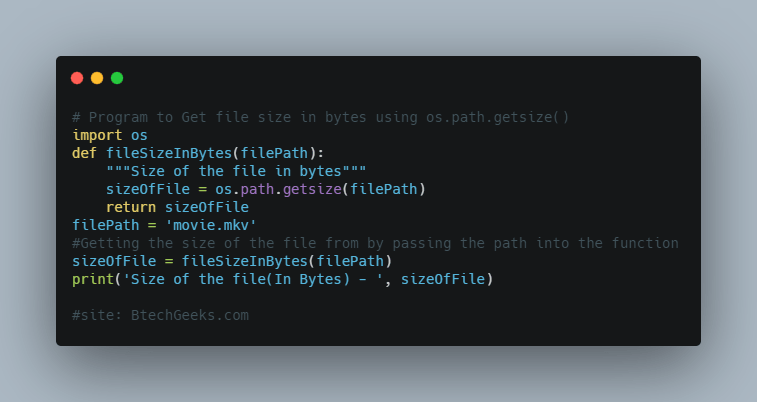Source Code:

# Program to Get file size in bytes using os.path.getsize()

import os
def fileSizeInBytes(filePath):
"""Size of the file in bytes"""
sizeOfFile = os.path.getsize(filePath)
return sizeOfFile
filePath = 'movie.mkv'
#Getting the size of the file from by passing the path into the function
sizeOfFile = fileSizeInBytes(filePath)
print('Size of the file(In Bytes) - ', sizeOfFile)

Output :
Size of the file(In Bytes) -  349016027

#### Method-2: Get file size in bytes using os.stat().st_size

We can also find the size of the file using a stats( ) function available in Python’s OS module. It takes the file path as an argument and returns an object containing the file details.

Syntax:

os.stat(path, *, dir_fd=None, follow_symlinks=True)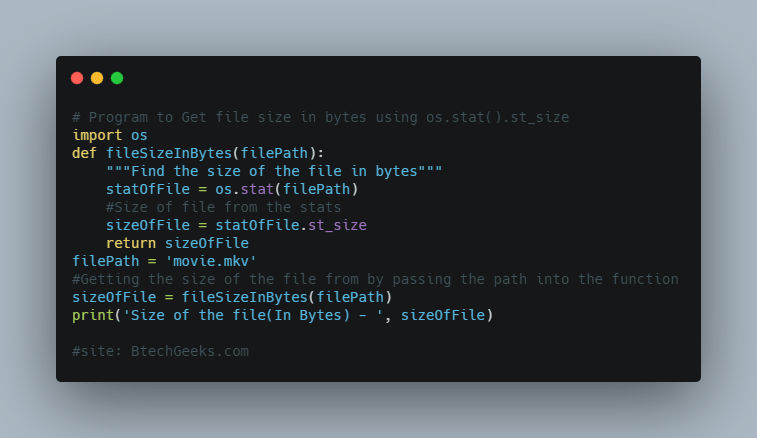Source Code:

# Program to Get file size in bytes using os.stat().st_size

import os
def fileSizeInBytes(filePath):
"""Find the size of the file in bytes"""
statOfFile = os.stat(filePath)
#Size of file from the stats
sizeOfFile = statOfFile.st_size
return sizeOfFile
filePath = 'movie.mkv'
#Getting the size of the file from by passing the path into the function
sizeOfFile = fileSizeInBytes(filePath)
print('Size of the file(In Bytes) - ', sizeOfFile)

Output :
Size of the file(In Bytes) -  349016027

#### Method-3: Get file size in bytes using pathlib.Path.stat().st_size

# program to Get file size in bytes using pathlib.Path.stat().st_size

from pathlib import Path
def fileSizeInBytes(filePath):
"""Size of the file in bytes"""
fileObj = Path(filePath)
#Size of file from the stats
sizeOfFile = fileObj.stat().st_size
return sizeOfFile
filePath = 'movie.mkv'
#Getting the size of the file from by passing the path into the function
sizeOfFile = fileSizeInBytes(filePath)
print('Size of the file(In Bytes) - ', sizeOfFile)

Output :
Size of the file(In Bytes) -  349016027

#### Method-4: Get file size in human-readable units like kilobytes (KB), Megabytes (MB), or GigaBytes (GB)

We can get the file sizes using the above method but we can not convert them to KB, MB, GB, TB directly so we have to create a function. The function would convert the units and so us the size of the file in our desired unit.

Here is a conversion table to switch from bytes to another unit of measurement :

 Units Number of bytes KiloBytes 1024 MegaBytes 1024*1024 = 1 048 576‬ GigaBytes 1024*1024*1024 = 1 073 741 824

et file size in human-readable units like kilobytes (KB), Megabytes (MB), or GigaBytes (GB

#Program to Get file size in human-readable units like KB, MB, GB, TB

import enum
import os

class sizeUnit(enum.Enum):
#class to store the various units
BYTES = 1
KB = 2
MB = 3
GB = 4

def unitConvertor(sizeInBytes, unit):
#Cinverts the file unit
if unit == sizeUnit.KB:
return sizeInBytes/1024
elif unit == sizeUnit.MB:
return sizeInBytes/(1024*1024)
elif unit == sizeUnit.GB:
return sizeInBytes/(1024*1024*1024)
else:
return sizeInBytes

def fileSize(filePath, size_type):
"""File size in KB, MB and GB"""
size = os.path.getsize(filePath)
return unitConvertor(size, size_type)

filePath = 'movie.mkv'
sizeOfFile = fileSize(filePath,sizeUnit.BYTES)
print('Size of the file in - ', sizeOfFile)
#Printing the size of file in KB
sizeOfFile = fileSize(filePath,sizeUnit.KB)
print('Size of the file in KB - ', sizeOfFile)
#Printing the size of file in MB
sizeOfFile = fileSize(filePath,sizeUnit.MB)
print('Size of the file in MB - ', sizeOfFile)
#Printing the size of file in GB
sizeOfFile = fileSize(filePath,sizeUnit.GB)
print('Size of the file in GB - ', sizeOfFile)

Output :
Size of the file in -  349016027
Size of the file in KB -  340835.9638671875
Size of the file in MB -  332.8476209640503
Size of the file in GB -  0.32504650484770536

### Check if the file exists before checking for the size of the file

In case the file does not exist it will throw an error disrupting our program’s execution. So to handle that we can check if the file exists or not and if not then handle it by using the if-else block.

# Program to check if the file exists before checking for the size of the file

import os

def fileSizeInBytes(filePath):
""" Size of the file in bytes"""
sizeOfFile = os.path.getsize(filePath)
return sizeOfFile

#Passing a file name that does not exist into the program
fileName = 'nonExistentFile.txt'
if os.path.exists(fileName):
size = fileSizeInBytes(fileName)
print('Size of file in Bytes : ', size)
else:
print('File not there')

Output :
File not there## Python Numpy: flatten() vs ravel() | Difference between the ravel numpy and flatten numpy functions

In this tutorial, python beginners and experts can easily learn about the difference between numpy ravel and flatten functions. The main aim of both numpy.ravel() and numpy.flatten() functions are the same i.e. to flatten a numpy array of any shape. Before learning about flatten ravel differences, let’s take a look at the basic overview of both the numpy functions from here.

## numpy.ravel()

ravel() is a built-in function in the module of numpy that returns a view of the original array and also returns a flattened 1D view of the numpy array object. Moreover, it accepts an array-like element as a parameter.

### Syntax of the ravel() function in numpy:

numpy.ravel(a, order='C')


## numpy.ndarray.flatten()

Flatten always returns a copy. The function of the flatten() is a faster member function of the numpy array where it returns a flattened 1D copy of the numpy array object.

### Syntax of Numpy flatten() function:

ndarray.flatten(order='C')


Let’s discuss some of the differences between the numpy ravel & flatten function:

## Differences between ravel() & flatten()

First of all, import the numpy module,

import numpy as np

A quick view about the difference between flatten and ravel functions in numpy is furnished below:

a.flatten()

(i) Return copy of the original array

(ii) If you modify any value of this array value of the original array is not affected.

(iii) Flatten() is comparatively slower than ravel() as it occupies memory.

(iv) Flatten is a method of a ndarray object.

a.ravel()

(i) Return only reference/view of original array

(ii) If you modify the array you would notice that the value of the original array also changes.

(iii) Ravel is faster than flatten() as it does not occupy any memory.

(iv) Ravel is a library-level function.

For in-depth knowledge on the difference between flatten and ravel numpy functions, kindly check out the further sections without any fail.

### Difference 1: Performance: Copy vs view

Thenumpy.ndarray.flatten()function returns a flatten copy of numpy array object only, whereas numpy.ravel() returns a flattened view of the input array if possible.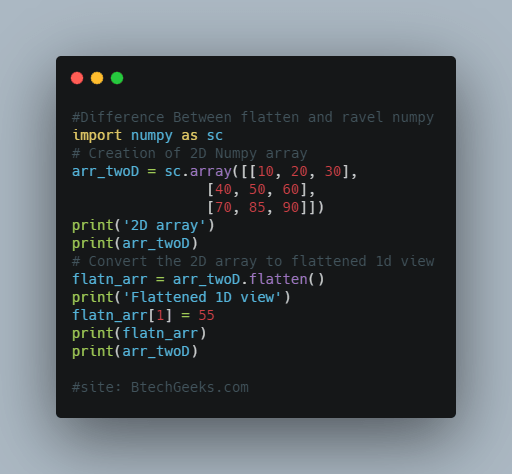import numpy as sc
# Creation of 2D Numpy array
arr_twoD = sc.array([[10, 20, 30],
[40, 50, 60],
[70, 85, 90]])
print('2D array')
print(arr_twoD)
# Convert the 2D array to flattened 1d view
flatn_arr = arr_twoD.flatten()
print('Flattened 1D view')
flatn_arr = 55
print(flatn_arr)
print(arr_twoD)

Output :
2D array
[[10 20 30]
[40 50 60]
[70 85 90]]
Flattened 1D view
[10 55 30 40 50 60 70 85 90]
[[10 20 30]
[40 50 60]
[70 85 90]]

Here we can successfully change 2nd element of the numpy array that is reflected in the 1D numpy array. But the original 2D array is unaffected which denotes that flatten() returns a copy of the input array.

Let’s try using numpy.ravel() to convert 2D numpy array to 1D array

ravel() returns a flattened view of the numpy array object if possible. When there is any change done in the view object it will also get reflected in the original numpy array.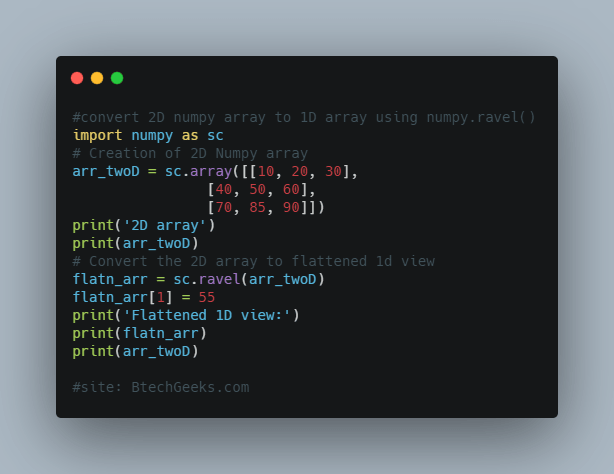import numpy as sc
# Creation of 2D Numpy array
arr_twoD = sc.array([[10, 20, 30],
[40, 50, 60],
[70, 85, 90]])
print('2D array')
print(arr_twoD)
# Convert the 2D array to flattened 1d view
flatn_arr = sc.ravel(arr_twoD)
flatn_arr = 55
print('Flattened 1D view:')
print(flatn_arr)
print(arr_twoD)

Output :
2D array
[[10 20 30]
[40 50 60]
[70 85 90]]
Flattened 1D view:
[10 55 30 40 50 60 70 85 90]
[[10 55 30]
[40 50 60]
[70 85 90]]

We can check of the ravel()function returns a view object or not by using the base attribute of the returned object. If it is None then it returns to the original numpy array i.e. it returns a flattened array which is a view only.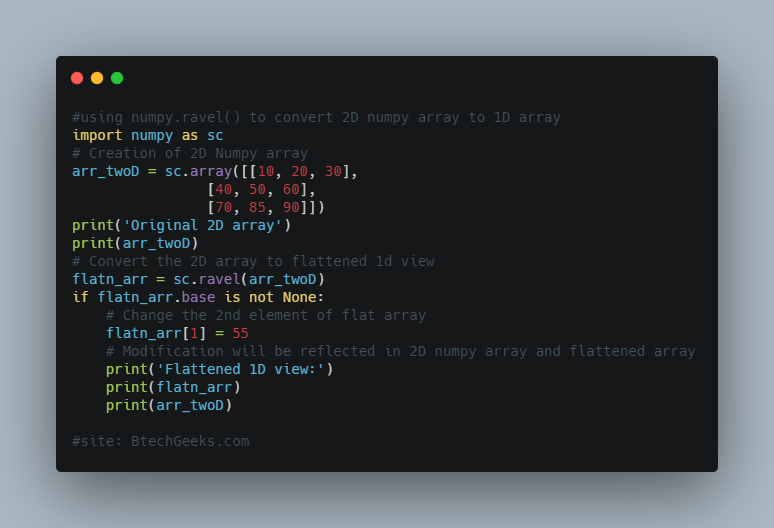import numpy as sc
# Creation of 2D Numpy array
arr_twoD = sc.array([[10, 20, 30],
[40, 50, 60],
[70, 85, 90]])
print('Original 2D array')
print(arr_twoD)
# Convert the 2D array to flattened 1d view
flatn_arr = sc.ravel(arr_twoD)
if flatn_arr.base is not None:
# Change the 2nd element of flat array
flatn_arr = 55
# Modification will be reflected in 2D numpy array and flattened array
print('Flattened 1D view:')
print(flatn_arr)
print(arr_twoD)

Output :
Original 2D array
[[10 20 30]
[40 50 60]
[70 85 90]]
Flattened 1D view:
[10 55 30 40 50 60 70 85 90]
[[10 55 30]
[40 50 60]
[70 85 90]]

So ravel() returns a view of the input array and hence here the performance of ravel() is better than flatten().

### Difference 2: Compatibility with other array-like sequences (list etc)

The ndarray.flatten()function can be used to flatten a numpy array object only. In the case of a numpy.ravel()function being a built-in function, it accepts an array-like element, so we can even pass a list to it.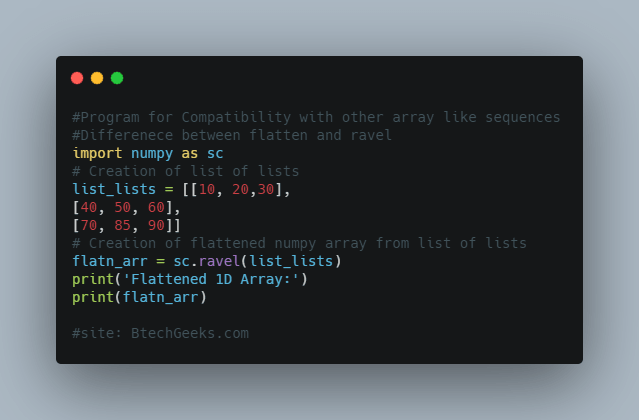import numpy as sc
# Creation of list of lists
list_lists = [[10, 20,30],
[40, 50, 60],
[70, 85, 90]]
# Creation of flattened numpy array from list of lists
flatn_arr = sc.ravel(list_lists)
print('Flattened 1D Array:')
print(flatn_arr)
Output :
Flattened 1D Array:
[10 20 30 40 50 60 70 85 90]

While this is not feasible with ndarray.flatten() function.

### Conclusion on A view of the original array using numpy ravel

Therefore, to conclude in the end there are 2 main differences between the ndarray.flatten() and numpy.ravel() function,

1. ravel() function returns a view of the array if possible, where flatten() always returns a copy. Therefore the performance of ravel() is much better than flatten()
2. ravel() function can accept other array-like elements like lists etc. Although, flatten() can work with numpy arrays only.## np.delete(): Remove items/rows/columns from Numpy Array | How to Delete Rows/Columns in a Numpy Array?

In this tutorial, we will discuss about how we can remove items/rows/columns from the Numpy array. We can remove elements from a NumPy array by index position using numpy.delete( ). Get to know the syntax of numpy.delete() function and learn about all its parameters with a clear explanation. Refer to the further modules to be aware of procedures on how to remove or delete an element or multiple elements in rows/columns of 1D and 2D Arrays by its Index Position.

## How to remove items/rows/columns from Numpy array using np.delete() in Python?

Python provides a method to delete elements from the numpy array depending on the index position and its syntax is provided below.

numpy.delete( )

Python’s NumPy library has a method to delete elements. It is done by using the np.delete( ) function.

Syntax:

numpy.delete(arr, obj, axis=None)

Where,

• arr: Array from which elements are to be deleted
• obj: Index position or positions from which elements are to be deleted
• axis: The axis along which we want to delete (1 means delete along columns, 0 means delete along the rows, if None then the array is flattened out and then the elements are to be deleted)

It returns a copy of the array with the required elements deleted.

### Delete an Element in 1D Numpy Array by its Index position

Let’s see the implementation of it.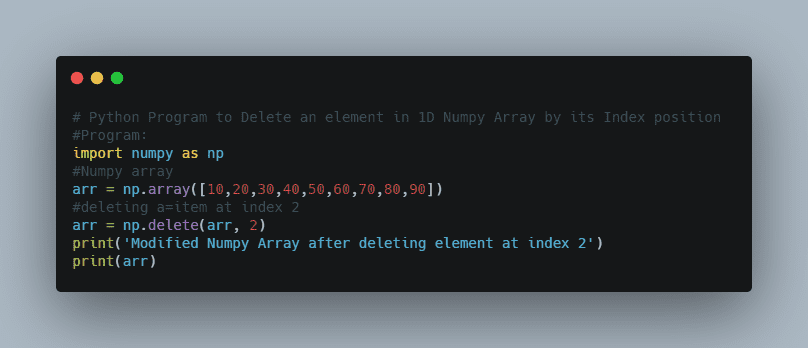#Program :

import numpy as np
#Numpy array
arr = np.array([10,20,30,40,50,60,70,80,90])
#deleting a=item at index 2
arr = np.delete(arr, 2)
print('Modified Numpy Array after deleting element at index 2')
print(arr)

Output :
Modified Numpy Array after deleting element at index 2
[10 20 40 50 60 70 80 90]

### Delete multiple elements in 1D Numpy Array by its Index position

Let’s see the implementation of it.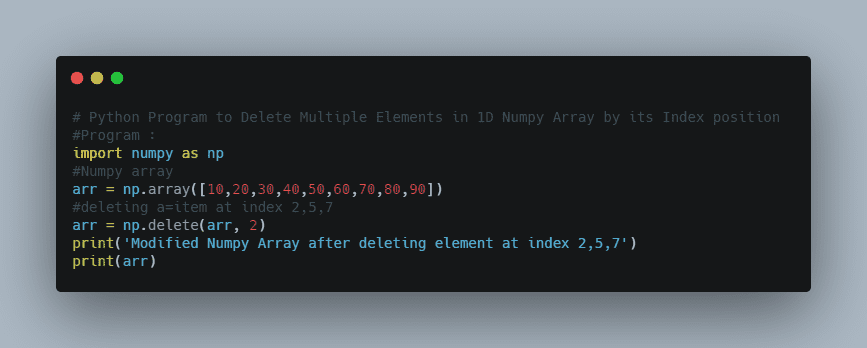#Program :

import numpy as np
#Numpy array
arr = np.array([10,20,30,40,50,60,70,80,90])
#deleting a=item at index 2,5,7
arr = np.delete(arr, 2)
print('Modified Numpy Array after deleting element at index 2,5,7')
print(arr)
Output :
Modified Numpy Array after deleting element at index  2,5,7
[10 20 40 50 70 90]

## Deleting rows & columns from a 2D Numpy Array

### Delete a column in 2D Numpy Array by its column number

if we want to delete a column from a 2D numpy array using np.delete() then we have to pass the axis=1 along with numpy array and index of column.

Let’s see the implementation of it.

#program

import numpy as np

arr2d = np.array([[10,20,30],
[40,50,60],
[70,80,90]])
#deleting elements at column 1
arr = np.delete(arr2d, 1, axis=1)
print('Modified Numpy Array by deleting element at index position 2,5,7')
print(arr)

Output :
Modified Numpy Array by deleting element at index position 2,5,7
[[10 30]
[40 60]
[70 90]]

### Delete multiple columns in 2D Numpy Array by its column number

To delete multiple columns pass axis=1 and list of column numbers to be deleted along with numpy array to the function.

Let’s see the implementation of it.

#Program :

import numpy as np

arr2D = np.array([[11 ,12, 13, 11],
[21, 22, 23, 24],
[31, 32, 33, 34]])
arr2D = np.delete(arr2D, [2,3], axis=1)
print('Modified 2D Numpy Array by removing columns at index 2 and 3')
print(arr2D)

Output :
Modified 2D Numpy Array by removing columns at index 2 and 3
[[11 12]
[21 22]
[31 32]]

### Delete a row in 2D Numpy Array by its row number

To delete a row from a 2D numpy array using np.delete() we need to pass the axis=0 along with numpy array and the row index.

Let’s see the implementation of it.#Program :

import numpy as np
arr2D = np.array([[11 ,12, 13, 11], [21, 22, 23, 24], [31, 32, 33, 34]])
arr2D = np.delete(arr2D, 0, axis=0)
print('Modified 2D Numpy Array by removing rowss at index 0')
print(arr2D)
Output :
Modified 2D Numpy Array by removing rowss at index 0
[[21 22 23 24]
[31 32 33 34]]

### Delete multiple rows in 2D Numpy Array by its row number

To delete multiple rows pass axis=0 and list of row numbers to be deleted along with numpy array to np.delete()

#Program :

import numpy as np

arr2D = np.array([[11 ,12, 13, 11],
[21, 22, 23, 24],
[31, 32, 33, 34]])
arr2D = np.delete(arr2D, [1, 2], axis=0)
print('Modified 2D Numpy Array by removing rows at index 1 and 2')
print(arr2D)

Output :
Modified 2D Numpy Array by removing rows at index 1 and 2
[[11 12 13 11]]

### Delete specific elements in 2D Numpy Array by its index position

When we don’t mention axis value, the default value is none which means the array gets flattened. After that, we use np.delete() to delete elements from rows and columns. The function will return a flattened array without the deleted rows and column elements.

#program :

import numpy as np

arr2D = np.array([[11 ,12, 13, 11],
[21, 22, 23, 24],
[31, 32, 33, 34]])
arr2D = np.delete(arr2D, 2)
print('Modified 2D Numpy Array by removing element at row 0 column 2')
print(arr2D)

Output :
Modified 2D Numpy Array by removing element at row 0 column 2
[11 12 11 21 22 23 24 31 32 33 34]## How to save Numpy Array to a CSV File using numpy.savetxt() in Python? | Savetxt Function’s Working in Numpy with Examples

NumPy arrays are very essential data structures for working with data in Python, machine learning models. Python’s Numpy module provides a function to save a numpy array to a txt file with custom delimiters and other custom options. In this tutorial, we will discuss the procedure of how to save Numpy Array to a CSV File with clear steps.

## numpy.savetxt() Function

The numpy.savetxt() function is the counterpart of the NumPy loadtxt() function and can save arrays in delimited file formats such as CSV. Save the array we created with the following function call:

Synatx : numpy.savetxt(fname, array_name, fmt='%.18e', delimiter=' ', newline='\n', header='', footer='', comments='# ', encoding=None)

Where,

• fname: If the filename ends in .gz, the file is automatically saved in compressed gzip format. The loadtxt() function can understand gzipped files transparently.
• arr_name: It indicates data to be saved like 1D or 2D numpy array.
• fmt: It refers to a formatting pattern or sequence of patterns, which will be used while saving elements to file.
• delimiter: It is optional, refers to string or character to be used as element separator
• newline: It is optional, refers to string or character to be used as line separator
• header: It refers to a string that is written at the beginning of the file.
• footer: It refers to a string that to be written at the end of the txt file.
• comments: It refers to a custom comment marker, where the default is ‘#’. It will be pre-appended to the header and footer.

## How to save Numpy Array to a CSV File using numpy.savetxt() in Python?

One of the most common file formats for storing numerical data in files is the comma-separated variable format or CSV in Short. Usually, input data are stored in CSV Files as it is one of the most convenient ways for storing data.

Savetxt function is used to save Numpy Arrays as CSV Files. The function needs a filename and array as arguments to save an array to CSV File. In addition, you need to mention the delimiter; for separating each variable in the file or most commonly comma. You can set via the “delimiter” argument.

### Example Program on How to Save a Numpy Array to a CSV File

#Program :

import numpy as np
def main():
# Numpy array created with a list of numbers
array1D = np.array([9, 1, 23, 4, 54, 7, 8, 2, 11, 34, 42, 3])
print('Real Array : ', array1D)
print('<** Saved 1D Numpy array to csv file **>')
# Save Numpy array to csv
np.savetxt('array.csv', [array1D], delimiter=',', fmt='%d')
print('*** Saving 1D Numpy array to csv file with Header and Footer ***')
# Saving Numpy array to csv with custom header and footer
np.savetxt('array_hf.csv', [array1D], delimiter=',', fmt='%d' , header='A Sample 2D Numpy Array :: Header', footer='This is footer')

print('*** Saving 2D Numpy array to csv file ***')
# A 2D Numpy array list of list created
array2D = np.array([[111, 11, 45, 22], [121, 22, 34, 14], [131, 33, 23, 7]])
print('2D Numpy Array')
print(array2D)
# Saving 2D numpy array to csv file
np.savetxt('2darray.csv', array2D, delimiter=',', fmt='%d')
# Saving 2nd column of 2D numpy array to csv file
np.savetxt('2darray_column.csv', [array2D[:,1]], delimiter=',', fmt='%d')
# Saving 2nd row of 2D numpy array to csv file
np.savetxt('2darray_row.csv', [array2D ], delimiter=',', fmt='%d')

# Creating the type of a structure
dtype = [('Name', (np.str_, 10)), ('Marks', np.float64), ('GradeLevel', np.int32)]
#Creating a Strucured Numpy array
structuredArr = np.array([('Sam', 33.3, 3), ('Mike', 44.4, 5), ('Aadi', 66.6, 6), ('Riti', 88.8, 7)], dtype=dtype)
print(structuredArr)
# Saving 2D numpy array to csv file
if __name__ == '__main__':
main()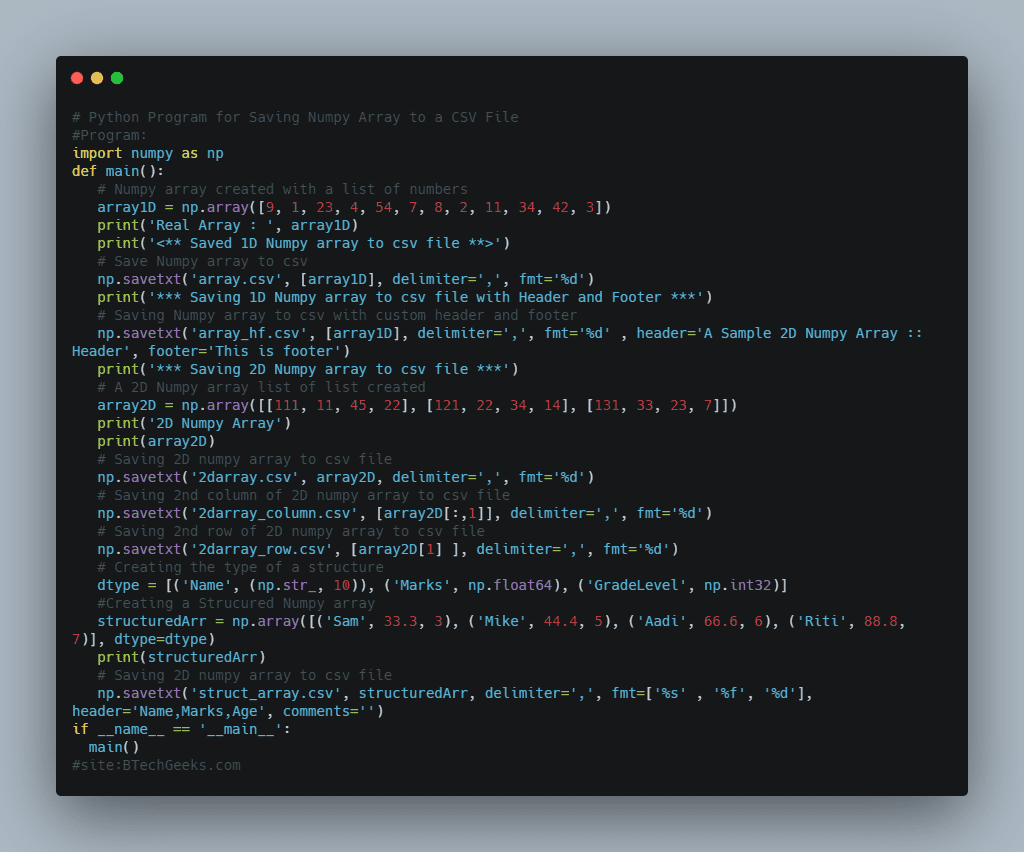Output:

Real Array :  [ 9  1 23  4 54  7  8  2 11 34 42  3]
<** Saved 1D Numpy array to csv file **>
<** Saved 1D Numpy array to csv file with custom  Header and Footer **>
<** Save 2D Numpy array to csv file **>
* 2D Numpy Array *
[[111  11  45  22]
[121  22  34  14]
[131  33  23   7]]
[('Rags', 33.3, 3) ('Punit', 44.4, 5) ('Drishti', 66.6, 6)  ('Ritu', 88.8, 7)]

The Passed Delimeter ‘,’ will change to CSV Format. In addition, the format string %d passed will store the elements as integers. By default, it will store numbers in float format. Keep in mind that if you don’t mention [] around numpy array to change it to list while passing numpy.savetxt() comma delimiter willn’t work and uses ‘\n’ by default. Thus, surrounding array by [] i.e. [arr] is mandatory.

### Save 1D Numpy array to CSV file with Header and Footer

# Save Numpy array to csv with custom header and footer
np.savetxt('array_hf.csv', [arr], delimiter=',', fmt='%d' , header='A Sample 2D Numpy Array :: Header', footer='This is footer')

Usually, By default comments in both the header and footer are pre-appended by ‘#’. To change this we can pass the parameter comments like comments=’@’.

### Final Words

Numpy savetxt can be an extremely helpful method for saving an array to CSV File. If you want to manipulate or change the existing data set this can be a great method. If you have any queries don’t hesitate to ask us via comment box so that we can get back to you at the soonest possible. Bookmark our site for the latest updates on Python, Java, C++, and Other Programming Languages.## Python: Check if a Process is Running by Name and find its Process ID (PID) | How to find the PID of a Running Process?

Do you want to determine whether a process is running or not by name and find its Process ID as well? Then, this is the right place as this article will shed light on you completely on how to check if a process is running or not using Name. We have provided enough examples for finding the Process ID PID by Name for better understanding. You can visit our site online to seek guidance or simply copy-paste the code from our portal and try to apply this knowledge and write a code on your own.

## How to Check if there exists a Process running by Name and find its Process ID (PID)?

In this article, we will discuss finding a running process PIDs by name using psutil.

psutil library can be installed by using

pip install psutil

### Determine if Process is Running or Not

Follow the simple guidelines for checking if a process is running or not. They are as such

• def findProcessIdByName(processName):
• for proc in psutil. process_iter():
• pinfo = proc. as_dict(attrs=[‘pid’, ‘name’, ‘create_time’])
• if processName. lower() in pinfo[‘name’]. lower() :
• except (psutil.NoSuchProcess, psutil.AccessDenied , psutil.ZombieProcess) :

To check if a process is running or not we will iterate over all the running processes using psutil.process_iter() and during iteration match the process name.

So let’s see this example to check chrome process is running or not.

import psutil
def checkProcessRunning(processName):
# Checking if there is any running process that contains the given name processName.
#Iterate over the all the running process
for proc in psutil.process_iter():
try:
# Check if process name contains the given name string.
if processName.lower() in proc.name().lower():
return True
except (psutil.NoSuchProcess, psutil.AccessDenied, psutil.ZombieProcess):
pass
return False;

# Checking if any chrome process is running or not.
if checkProcessRunning('chrome'):
print('Yes chrome process was running')
else:
print('No chrome process was running')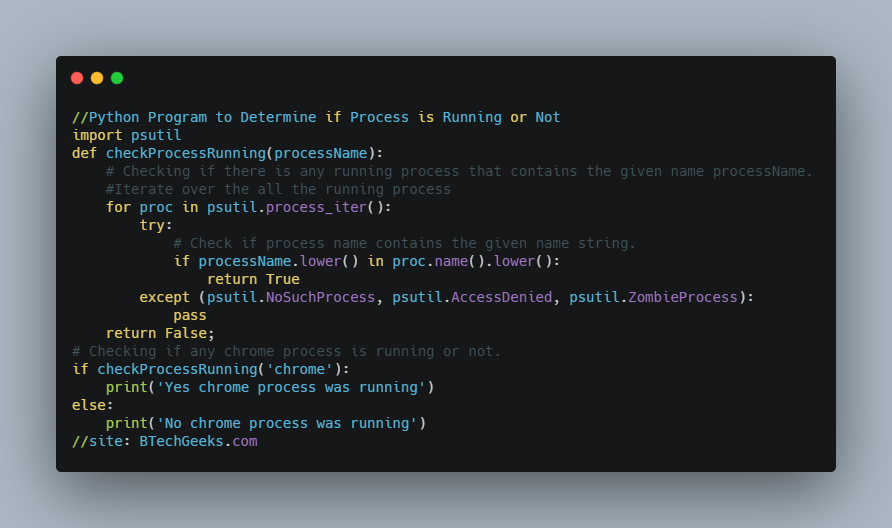Output:

No chrome process was running

As in my system chrome instances are not running it will return False.

### Finding the Process ID(PID) by Name?

In the above program, we checked any chrome instances were running or not. But in this example, we will see how to get the Process ID of a running ‘chrome.exe’ process. For that we will iterate over all the running processes and during iteration for each process whose name contains the given string, we will keep its information in a list. The following code helps you find the PID of a running process by Name.

import psutil
def findProcessIdByName(processName):
# Here is the list of all the PIDs of a all the running process
# whose name contains the given string processName
listOfProcessObjects = []
#Iterating over the all the running process
for proc in psutil.process_iter():
try:
pinfo = proc.as_dict(attrs=['pid', 'name', 'create_time'])
# Checking if process name contains the given name string.
if processName.lower() in pinfo['name'].lower() :
listOfProcessObjects.append(pinfo)
except (psutil.NoSuchProcess, psutil.AccessDenied , psutil.ZombieProcess) :
pass
return listOfProcessObjects;

# Finding PIDs od all the running instances of process
# which contains 'chrome' in it's name
listOfProcessIds = findProcessIdByName('chrome')
if len(listOfProcessIds) > 0:
print('Process Exists | PID and other details are')
for elem in listOfProcessIds:
processID = elem['pid']
processName = elem['name']
processCreationTime =  time.strftime('%Y-%m-%d %H:%M:%S', time.localtime(elem['create_time']))
print((processID ,processName,processCreationTime ))
else :
print('No Running Process found with this text')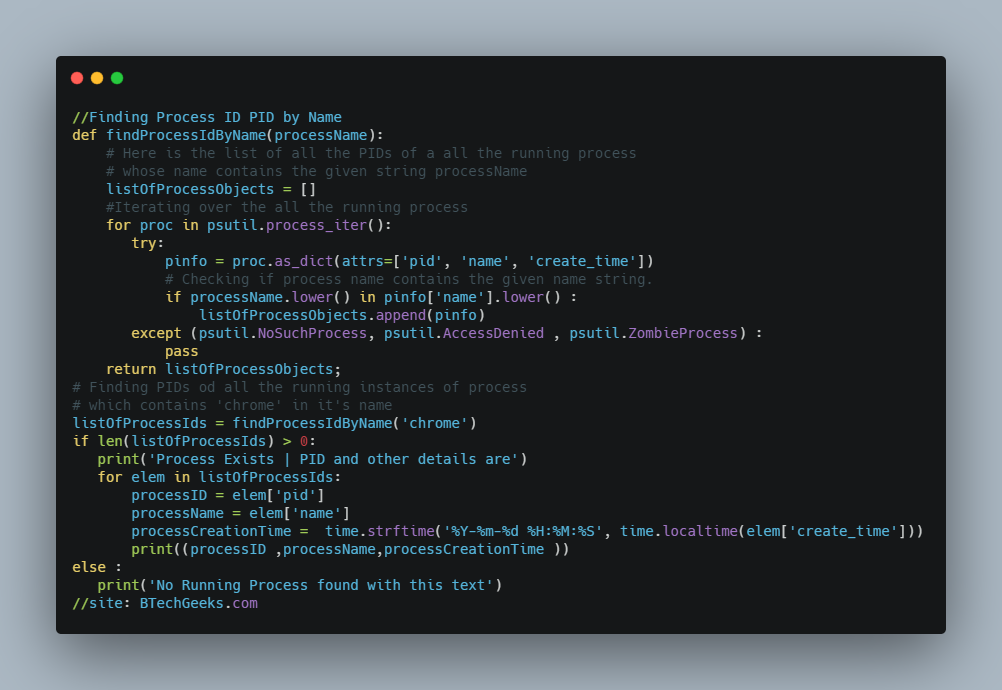Now, the contents of the list

(2604, 'chrome.exe', '2018-11-10 19:12:13')
(4276, 'chrome.exe', '2018-11-10 19:12:14')
(9136, 'chrome.exe', '2018-11-10 19:12:14')
(9616, 'chrome.exe', '2018-11-10 19:43:41')
(12904, 'chrome.exe', '2018-11-10 19:12:13')
(13476, 'chrome.exe', '2018-11-10 20:03:04')
(15520, 'chrome.exe', '2018-11-10 20:02:22')

This can also be done using a single line using list comprehension  i.e

# Finding the PIDs of all the running instances of process that contains 'chrome' in it's name
procObjList = [procObj for procObj in psutil.process_iter() if 'chrome' in procObj.name().lower() ]

And the same output will come like

psutil.Process(pid=2604, name='chrome.exe', started='19:12:13')
psutil.Process(pid=4276, name='chrome.exe', started='19:12:14')
psutil.Process(pid=9136, name='chrome.exe', started='19:12:14')
psutil.Process(pid=9616, name='chrome.exe', started='19:43:41')
psutil.Process(pid=12904, name='chrome.exe', started='19:12:13')
psutil.Process(pid=13476, name='chrome.exe', started='20:03:04')
psutil.Process(pid=15520, name='chrome.exe', started='20:02:22')

Related Programs:

## Deleting elements from a NumPy Array by value or conditions in Python.

In this article we will discuss about how to delete elements from a NumPy Array by based on matching values or multiple conditions.

### Remove all occurrences of an element with given value from numpy array :

Suppose we have a NumPy array and we want to delete all the occurrences of that particular element from that Numpy array.

#Program :

import numpy as np
# numpy array created from a list
arr = np.array([40,50,60,70,80,90,40,10,20,40])
print('Numpy Array before deleting all occurrences of 40 :')
print(arr)

# Removing all occurrences of elements with value 40 from numpy array
arr = arr[arr != 40]
print('Modified Numpy Array after deleting all occurrences of 40 :')
print(arr)

Output :
Numpy Array before deleting all occurrences of 40 :
[40 50 60 70 80 90 40 10 20 40]
Modified Numpy Array after deleting all occurrences of 40 :
[50 60 70 80 90 10 20]

The condition arr != 40 returns a bool array of same size as arr with value True at places where value is not equal to 40 and returns False at other places. Like this

[ False True True True True True False True True False]

When we will pass it to [] of numpy array arr then it will select the elemnts whose value is True. Means it will return the elements from arr which are not  equal to 40.

You can also delete column using numpy delete column tutorial.

### Delete elements in Numpy Array based on multiple conditions :

Like above example, it will create a bool array using multiple conditions on numpy array and when it will be passed to [] operator of numpy array to select the elements then it will return a copy of the numpy array satisfying the condition suppose (arr > 40) & (arr < 80) means elements greater than 40 and less than 80 will be returned.

#Program :

import numpy as np
# numpy array created from a list
arr = np.array([40,50,60,70,80,90,40,10,20,40])
print('Numpy Array before deleting any element :')
print(arr)

# Remove all occurrences of elements below 40 & greater than 80
# Means keeping elements greater than 40 and less than 80
arr = arr[ (arr > 40) & (arr < 80) ]
print('Modified Numpy Array after deleting elements :')
print(arr)

Output :
Numpy Array before deleting any element :
[40 50 60 70 80 90 40 10 20 40]
Modified Numpy Array after deleting elements :
[50 60 70]

### Delete elements by value or condition using np.argwhere() & np.delete() :

By using np.argwhere() & np.delete()  we can also delete any elements.

#Program :

import numpy as np
# numpy array created from a list
arr = np.array([40,50,60,70,80,90,40,10,20,40])
print('Numpy Array before deleting any element :')
print(arr)

# deleting all occurrences of element with value 40
arr = np.delete(arr, np.argwhere(arr == 40))
print('Modified Numpy Array after deleting all occurrences of 40')
print(arr)

Output :
Numpy Array before deleting all occurrences of 40 :
[40 50 60 70 80 90 40 10 20 40]
Modified Numpy Array after deleting all occurrences of 40 :
[50 60 70 80 90 10 20]

In the above example np.delete() function will delete the element and np.argwhere() function will detect the index.
Means the condition arr == 40 will return a bool array like

[True False False False False False True False False True]

Where the condition arr == 40 matches it returns True and if condition fails it returns False. And when the bool array will be passed inside the function np.argwhere() then it will return the index positions where the values are True.

i.e

[ 

]

These are the indices which represents the value 40.

When this index positions will be passed inside np.delete() function then the element present at those index positions will be deleted.

### Delete elements by multiple conditions using np.argwhere() & np.delete() :

The concept is same like above only the difference is the elements will be deleted based on multiple conditions.

So, let’s see the implementation of it.

#Program :

import numpy as np
# numpy array created from a list
arr = np.array([40,50,60,70,80,90,40,10,20,40])
print('Numpy Array before deleting any element :')
print(arr)

#It will delete all the elements which are greater than 40 and less than 80
arr = np.delete(arr, np.argwhere( (arr >= 40) & (arr <= 80) ))
print('Modified Numpy Array after deleting :')
print(arr)

Output :
Numpy Array before deleting any element :
[40 50 60 70 80 90 40 10 20 40]
Modified Numpy Array after deleting elements :
[90 10 20]## Placement new operator and it’s need

### Placement new operator :

placement new operator we use to pass a memory address to new as a parameter. This memory is used by the placement new operator to create the object and also to call the constructor on it and then returning the same passed address.

### Need of placement new operator :

As we know when we create any object using new operator, then the memory is allocated on the heap.

int * ptr = new int;

But, while working sometimes we need to create an object dynamically for which some specific memory location will be allocated.

For example, we do not want new memory to be allocated on heap rather it needs to be allocated on a given memory address. Actually this scenario comes when we work on any embedded product or with shared memory. So, for this requirement we use placement new operator.

Below is an example code to achieve this :

// Program

#include <iostream>
#include <cstdlib>
#include <new>

int main()
{
// Here memory will not be allocated on heap.
int * space = new int;
// It will use passed spacer to allocate the memory
int * ptr = new(space) int;
*ptr = 7;
std::cout<<(*ptr)<<std::endl;
delete [] buffer;
return 0;
}## Adding an element to Numpy Array in Python

In this article we are going to discuss 3 different ways to add element to the numpy array.

Let’s see one by one method.

### Method-1 : By using append() method :

In numpy module of python there is a function numpy.append() which can be used to add an element. We need to pass the element as the argument of the function.

Let’s take a  example where an array is declared first and then we used the append() method to add more elements  to the array.

import numpy as np
arr = np.array([12, 24, 36])
new_Arr = np.append (arr, [48,50,64])
print(‘ array : ’,arr)
print(‘result = : ’ ,new_Arr)

Output :
array : [ 12  24  36 ]
result = : [ 12   24   36  48   50   64 ]

We can also insert a column by the use of  append() method .

Let’s take an example below where we created a 2-Darray and we have to  insert two columns at a specific place.

import numpy as np
arr1= np.array([[12, 24, 36], [48, 50, 64]])
arr2 = np.array([, ])
new_Array = np.append(arr1,arr2, axis = 1)
print(' array : ',arr1)
print(' result = : ', new_Array)

Output :
array : [ [ 12  24  36  ]
[ 48  50  64  ] ]
result  : [  [ 12  24  36  7  ]
[48  50  64   8  ]  ]

We can also insert a row  by the use of  append() method.

Let’s take the  example below where we created a 2-D array and we have to  inserte  a row to it.

import numpy as np
arr= np.array([[12, 24, 36], [48, 50, 62]])
new_Array = np.append(arr, [[70 ,80 ,90 ]], axis = 0)
print('array = ', arr )
print('result = ' ,new_Array)

Output :
array = [ [ 12  24   36   ]
[ 48  50    64   ] ]
result = [  [ 12   24   36   ]
[ 48   50  64   ]
[70   80   90 ] ]

### Method-2 : By using concatenate() method :

In numpy module of python there is a function numpy.concatenate() to join two or more arrays. To add a single element we need to encapsulate the single value in a sequence data structure like list pass it to the function.

import numpy as np
# Numpy Array of integers created
arr = np.array([1, 2, 6, 8, 7])
# Adding an element at the end of a numpy array
new_arr = np.concatenate( (arr,  ) )
print('array: ', new_arr)
print('result: ', arr)
Output :
array:[1, 2, 6, 8, 7]
result: [1, 2, 6, 8, 7,20]

Adding another array, see the below program

import numpy as np
arr1 = np.array([[10, 20], [30, 40]])
arr2 = np.array([[50, 60]])
new_Array= np.concatenate((arr1,arr2 ), axis=0)
print( 'First Array : ' ,arr1 )
print( 'Second Array : ' , arr2  )
print( 'concatenated Array : ' , new_Array )

Output :
First Array :   [[ 10  20 ]
[30  40 ]]
Second Array :  [[ 50  60 ] ]
Concatenated Array :     [[ 10   20 ]
[ 30   40 ]
[ 50    60 ]]

### Method-3 : By using insert() method :

In numpy module of python there is a function numpy.insert() to add an element at the end of the numpy array.

Below program is to add an elements to the array.

import numpy as np
arr= np.array (  [ 16, 33, 47, 59, 63 ,79 ])
# Here specified at index 1, so elemnt will eb replaced with new element
new_Array = np.insert(arr, 1, 20 )
print('The array : ', arr)
print ('result :',  new_Array)

Output :
array : [ 16  33  47  59  63  79 ]
result : [ 16  20  33  47  59  66  79 ]## Create a Numpy array of evenly spaced samples over a range using numpy.linspace()

In this article we will discus about how to create a Numpy array of evenly spaced samples over a range using numpy.linspace().

## Numpy.linspace()

Numpy module provides a function numpy.linspace() to create a evenly spaced samples over a specified interval i.e.

Syntax: numpy.linspace(start, stop, num=50, endpoint=True, retstep=False, dtype=None)
Where,
• start : Represents the start value of the range
• stop : Represents the end value of the range (Actually array doesn’t include this value but it acts as an end point)
• num : Represents the number of samples to be generated (If it is not provided then optional value is taken as 50)
• dtype : Represents datatype of elements.

To use Numpy we have to include the below module i.e.

import numpy as np

Let’s see below two examples to understand the concept.

### Example-1 : Create 5 evenly spaced samples in interval [10, 50]

import numpy as np
# Create 5 evenly spaced samples in interval [10, 50]
temp_arr = np.linspace(10,50,5)
print(temp_arr)
Output :
[10. 20. 30. 40. 50.]
We can also specify the datatype as int32 by dtype.
import numpy as np
# Create 5 evenly spaced samples in interval [10, 50]
temp_arr = np.linspace(10,50,5,dtype=np.int32)
print(temp_arr)
Output :
[10 20 30 40 50]

### Example-2 : Get the Step size from numpy.linspace()

When we will pass retstep=True in numpy.linspace().

Then it will return step size between samples.
import numpy as np
# Create 5 evenly spaced samples in interval [10, 50]
temp_arr, step = np.linspace(10,50,5,dtype=np.int32,retstep=True)
print('Printing the Numpy Array contents: ')
print(temp_arr)
print('The step size between two samples  : ', step)
Output :
Contents of the Numpy Array :
[10 20 30 40 50]
Step size between two elements : 10.0## Creating Numpy Array of different shapes & initialize with identical values using numpy.full()

In this article we will see how we can create a numpy array of different shapes but initialized with identical values. So, let’s start the explore the concept to understand it well.

### numpy.full() :

Numpy module provides a function numpy.full() to create a numpy array of given shape and initialized with a given value.

Syntax : numpy.full(shape, given_value, dtype=None, order='C')

Where,

• shape : Represents shape of the array.
• given_value : Represents initialization value.
• dtype : Represents the datatype of elements(Optional).

But to use Numpy we have to include following module i.e.

import numpy as np
Let’s see the below example to understand the concept.

### Example-1 : Create a 1D Numpy Array of length 8 and all elements initialized with value 2

Here array length is 8 and array elements to be initialized with 2.

Let’s see the below the program.

import numpy as np
# 1D Numpy Array created of length 8 & all elements initialized with value 2
sample_arr = np.full(8,2)
print(sample_arr)
Output :
[2 2 2 2 2 2 2 2]

### Example-2 : Create a 2D Numpy Array of 3 rows | 4 columns and all elements initialized with value 5

Here 2D array of row 3 and column 4 and array elements to be initialized with 5.

Let’s see the below the program.

import numpy as np
#Create a 2D Numpy Array of 3 rows & 4 columns. All intialized with value 5
sample_arr = np.full((3,4), 5)
print(sample_arr)
Output :
[[5 5 5 5]
[5 5 5 5]
[5 5 5 5]]

### Example-3 : Create a 3D Numpy Array of shape (3,3,4) & all elements initialized with value 1

Here initialized value is 1.

Let’s see the below the program.

import numpy as np
# Create a 3D Numpy array & all elements initialized with value 1
sample_arr = np.full((3,3,4), 1)
print(sample_arr)
Output :

[[[1 1 1 1]
[1 1 1 1]
[1 1 1 1]]

[[1 1 1 1]
[1 1 1 1]
[1 1 1 1]]

[[1 1 1 1]
[1 1 1 1]
[1 1 1 1]]]

### Example-4 : Create initialized Numpy array of specified data type

Here, array length is 8 and value is 4 and data type is float.

import numpy as np
# 1D Numpy array craeted & all float elements initialized with value 4
sample_arr = np.full(8, 4, dtype=float)
print(sample_arr)
Output :
[4. 4. 4. 4. 4. 4. 4. 4.]